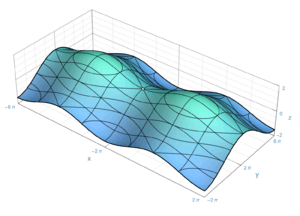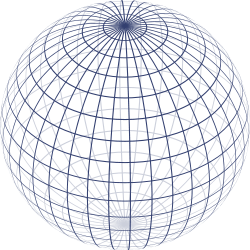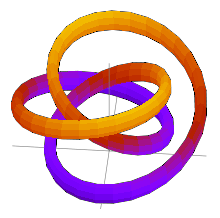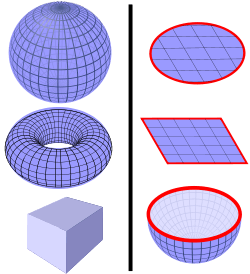# Surface (topology)An open surface with x-, y-, and z-contours shown.

In topology and differential geometry, a surface is a two-dimensional manifold, and, as such, may be an "abstract surface" not embedded in any Euclidean space. For example, the Klein bottle is a surface, which cannot be represented in the three-dimensional Euclidean space without introducing self-intersections (it cannot be embedded in the three dimensional Euclidean space).

## In general

Further information: Surface (mathematics)

In mathematics, a surface is a geometrical shape that resembles to a deformed plane. The most familiar examples arise as boundaries of solid objects in ordinary three-dimensional Euclidean space R3, such as spheres. The exact definition of a surface may depend on the context. Typically, in algebraic geometry, a surface may cross itself (and may have other singularities), while, in topology and differential geometry, it may not.

A surface is a two-dimensional space; this means that a moving point on a surface may move in two directions (it has two degrees of freedom). In other words, around almost every point, there is a coordinate patch on which a two-dimensional coordinate system is defined. For example, the surface of the Earth resembles (ideally) a two-dimensional sphere, and latitude and longitude provide two-dimensional coordinates on it (except at the poles and along the 180th meridian).

The concept of surface is widely used in physics, engineering, computer graphics, and many other disciplines, primarily in representing the surfaces of physical objects. For example, in analyzing the aerodynamic properties of an airplane, the central consideration is the flow of air along its surface.

## Definitions and first examples

A (topological) surface is a topological space in which every point has an open neighbourhood homeomorphic to some open subset of the Euclidean plane E2. Such a neighborhood, together with the corresponding homeomorphism, is known as a (coordinate) chart. It is through this chart that the neighborhood inherits the standard coordinates on the Euclidean plane. These coordinates are known as local coordinates and these homeomorphisms lead us to describe surfaces as being locally Euclidean.

In most writings on the subject, it is often assumed, explicitly or implicitly, that as a topological space a surface is also nonempty, second countable, and Hausdorff. It is also often assumed that the surfaces under consideration are connected.

The rest of this article will assume, unless specified otherwise, that a surface is nonempty, Hausdorff, second countable, and connected.

More generally, a (topological) surface with boundary is a Hausdorff topological space in which every point has an open neighbourhood homeomorphic to some open subset of the closure of the upper half-plane H2 in C. These homeomorphisms are also known as (coordinate) charts. The boundary of the upper half-plane is the x-axis. A point on the surface mapped via a chart to the x-axis is termed a boundary point. The collection of such points is known as the boundary of the surface which is necessarily a one-manifold, that is, the union of closed curves. On the other hand, a point mapped to above the x-axis is an interior point. The collection of interior points is the interior of the surface which is always non-empty. The closed disk is a simple example of a surface with boundary. The boundary of the disc is a circle.

The term surface used without qualification refers to surfaces without boundary. In particular, a surface with empty boundary is a surface in the usual sense. A surface with empty boundary which is compact is known as a 'closed' surface. The two-dimensional sphere, the two-dimensional torus, and the real projective plane are examples of closed surfaces.

The Möbius strip is a surface on which the distinction between clockwise and counterclockwise can be defined locally, but not globally. In general, a surface is said to be orientable if it does not contain a homeomorphic copy of the Möbius strip; intuitively, it has two distinct "sides". For example, the sphere and torus are orientable, while the real projective plane is not (because the real projective plane with one point removed is homeomorphic to the open Möbius strip).

In differential and algebraic geometry, extra structure is added upon the topology of the surface. This added structures can be a smoothness structure (making it possible to define differentiable maps to and from the surface), a Riemannian metric (making it possible to define length and angles on the surface), a complex structure (making it possible to define holomorphic maps to and from the surface — in which case the surface is called a Riemann surface), or an algebraic structure (making it possible to detect singularities, such as self-intersections and cusps, that cannot be described solely in terms of the underlying topology).

## Extrinsically defined surfaces and embeddingsA sphere can be defined parametrically (by x = r sin θ cos φ, y = r sin θ sin φ, z = r cos θ) or implicitly (by x2 + y2 + z2 r2 = 0.)

Historically, surfaces were initially defined as subspaces of Euclidean spaces. Often, these surfaces were the locus of zeros of certain functions, usually polynomial functions. Such a definition considered the surface as part of a larger (Euclidean) space, and as such was termed extrinsic.

In the previous section, a surface is defined as a topological space with certain properties, namely Hausdorff and locally Euclidean. This topological space is not considered a subspace of another space. In this sense, the definition given above, which is the definition that mathematicians use at present, is intrinsic.

A surface defined as intrinsic is not required to satisfy the added constraint of being a subspace of Euclidean space. It may seem possible for some surfaces defined intrinsically to not be surfaces in the extrinsic sense. However, the Whitney embedding theorem asserts every surface can in fact be embedded homeomorphically into Euclidean space, in fact into E4: The extrinsic and intrinsic approaches turn out to be equivalent.

In fact, any compact surface that is either orientable or has a boundary can be embedded in E3; on the other hand, the real projective plane, which is compact, non-orientable and without boundary, cannot be embedded into E3 (see Gramain). Steiner surfaces, including Boy's surface, the Roman surface and the cross-cap, are models of the real projective plane in E3, but only the Boy surface is an immersed surface. All these models are singular at points where they intersect themselves.

The Alexander horned sphere is a well-known pathological embedding of the two-sphere into the three-sphere.A knotted torus.

The chosen embedding (if any) of a surface into another space is regarded as extrinsic information; it is not essential to the surface itself. For example, a torus can be embedded into E3 in the "standard" manner (which looks like a bagel) or in a knotted manner (see figure). The two embedded tori are homeomorphic, but not isotopic: They are topologically equivalent, but their embeddings are not.

The image of a continuous, injective function from R2 to higher-dimensional Rn is said to be a parametric surface. Such an image is so-called because the x- and y- directions of the domain R2 are 2 variables that parametrize the image. A parametric surface need not be a topological surface. A surface of revolution can be viewed as a special kind of parametric surface.

If f is a smooth function from R3 to R whose gradient is nowhere zero, then the locus of zeros of f does define a surface, known as an implicit surface. If the condition of non-vanishing gradient is dropped, then the zero locus may develop singularities.

## Construction from polygons

Each closed surface can be constructed from an oriented polygon with an even number of sides, called a fundamental polygon of the surface, by pairwise identification of its edges. For example, in each polygon below, attaching the sides with matching labels (A with A, B with B), so that the arrows point in the same direction, yields the indicated surface.

Any fundamental polygon can be written symbolically as follows. Begin at any vertex, and proceed around the perimeter of the polygon in either direction until returning to the starting vertex. During this traversal, record the label on each edge in order, with an exponent of -1 if the edge points opposite to the direction of traversal. The four models above, when traversed clockwise starting at the upper left, yield

• sphere:• real projective plane:• torus:• Klein bottle:.

Note that the sphere and the projective plane can both be realized as quotients of the 2-gon, while the torus and Klein bottle require a 4-gon (square).

The expression thus derived from a fundamental polygon of a surface turns out to be the sole relation in a presentation of the fundamental group of the surface with the polygon edge labels as generators. This is a consequence of the Seifert–van Kampen theorem.

Gluing edges of polygons is a special kind of quotient space process. The quotient concept can be applied in greater generality to produce new or alternative constructions of surfaces. For example, the real projective plane can be obtained as the quotient of the sphere by identifying all pairs of opposite points on the sphere. Another example of a quotient is the connected sum.

## Connected sums

The connected sum of two surfaces M and N, denoted M # N, is obtained by removing a disk from each of them and gluing them along the boundary components that result. The boundary of a disk is a circle, so these boundary components are circles. The Euler characteristicof M # N is the sum of the Euler characteristics of the summands, minus two:The sphere S is an identity element for the connected sum, meaning that S # M = M. This is because deleting a disk from the sphere leaves a disk, which simply replaces the disk deleted from M upon gluing.

Connected summation with the torus T is also described as attaching a "handle" to the other summand M. If M is orientable, then so is T # M. The connected sum is associative, so the connected sum of a finite collection of surfaces is well-defined.

The connected sum of two real projective planes, P # P, is the Klein bottle K. The connected sum of the real projective plane and the Klein bottle is homeomorphic to the connected sum of the real projective plane with the torus; in a formula, P # K = P # T. Thus, the connected sum of three real projective planes is homeomorphic to the connected sum of the real projective plane with the torus. Any connected sum involving a real projective plane is nonorientable.

## Closed surfaces

"Open surface" redirects here. It is not to be confused with Free surface.

A closed surface is a surface that is compact and without boundary. Examples are spaces like the sphere, the torus and the Klein bottle. Examples of non-closed surfaces are: an open disk, which is a sphere with a puncture; a cylinder, which is a sphere with two punctures; and the Möbius strip.

### Classification of closed surfacesSome examples of orientable closed surfaces (left) and surfaces with boundary (right). Left: Some orientable closed surfaces are the surface of a sphere, the surface of a torus, and the surface of a cube. (The cube and the sphere are topologically equivalent to each other.) Right: Some surfaces with boundary are the disk surface, square surface, and hemisphere surface. The boundaries are shown in red. All three of these are topologically equivalent to each other.

The classification theorem of closed surfaces states that any connected closed surface is homeomorphic to some member of one of these three families:

1. the sphere;
2. the connected sum of g tori, for;
3. the connected sum of k real projective planes, for.

The surfaces in the first two families are orientable. It is convenient to combine the two families by regarding the sphere as the connected sum of 0 tori. The number g of tori involved is called the genus of the surface. The sphere and the torus have Euler characteristics 2 and 0, respectively, and in general the Euler characteristic of the connected sum of g tori is 2 2g.

The surfaces in the third family are nonorientable. The Euler characteristic of the real projective plane is 1, and in general the Euler characteristic of the connected sum of k of them is 2 k.

It follows that a closed surface is determined, up to homeomorphism, by two pieces of information: its Euler characteristic, and whether it is orientable or not. In other words, Euler characteristic and orientability completely classify closed surfaces up to homeomorphism.

Closed surfaces with multiple connected components are classified by the class of each of their connected components, and thus one generally assumes that the surface is connected.

### Monoid structure

Relating this classification to connected sums, the closed surfaces up to homeomorphism form a commutative monoid under the operation of connected sum, as indeed do manifolds of any fixed dimension. The identity is the sphere, while the real projective plane and the torus generate this monoid, with a single relation P # P # P = P # T, which may also be written P # K = P # T, since K = P # P. This relation is sometimes known as Dyck's theorem after Walther von Dyck, who proved it in (Dyck 1888), and the triple cross surface P # P # P is accordingly called Dyck's surface.

Geometrically, connect-sum with a torus (# T) adds a handle with both ends attached to the same side of the surface, while connect-sum with a Klein bottle (# K) adds a handle with the two ends attached to opposite sides of an orientable surface; in the presence of a projective plane (# P), the surface is not orientable (there is no notion of side), so there is no difference between attaching a torus and attaching a Klein bottle, which explains the relation.

### Surfaces with boundary

Compact surfaces, possibly with boundary, are simply closed surfaces with a finite number of holes (open discs that have been removed). Thus, a connected compact surface is classified by the number of boundary components and the genus of the corresponding closed surface – equivalently, by the number of boundary components, the orientability, and Euler characteristic. The genus of a compact surface is defined as the genus of the corresponding closed surface.

This classification follows almost immediately from the classification of closed surfaces: removing an open disc from a closed surface yields a compact surface with a circle for boundary component, and removing k open discs yields a compact surface with k disjoint circles for boundary components. The precise locations of the holes are irrelevant, because the homeomorphism group acts k-transitively on any connected manifold of dimension at least 2.

Conversely, the boundary of a compact surface is a closed 1-manifold, and is therefore the disjoint union of a finite number of circles; filling these circles with disks (formally, taking the cone) yields a closed surface.

The unique compact orientable surface of genus g and with k boundary components is often denotedfor example in the study of the mapping class group.

### Riemann surfaces

A closely related example to the classification of compact 2-manifolds is the classification of compact Riemann surfaces, i.e., compact complex 1-manifolds. (Note that the 2-sphere and the torus are both complex manifolds, in fact algebraic varieties.) Since every complex manifold is orientable, the connected sums of projective planes are not complex manifolds. Thus, compact Riemann surfaces are characterized topologically simply by their genus. The genus counts the number of holes in the manifold: the sphere has genus 0, the one-holed torus genus 1, etc.

### Non-compact surfaces

Non-compact surfaces are more difficult to classify. As a simple example, a non-compact surface can be obtained by puncturing (removing a finite set of points from) a closed manifold. On the other hand, any open subset of a compact surface is itself a non-compact surface; consider, for example, the complement of a Cantor set in the sphere, otherwise known as the Cantor tree surface. However, not every non-compact surface is a subset of a compact surface; two canonical counterexamples are the Jacob's ladder and the Loch Ness monster, which are non-compact surfaces with infinite genus.

A non-compact surface M has a non-empty space of ends E(M), which informally speaking describes the ways that the surface "goes off to infinity". The space E(M) is always topologically equivalent to a closed subspace of the Cantor set. M may have a finite or countably infinite number Nh of handles, as well as a finite or countably infinite number Np of projective planes. If both Nh and Np are finite, then these two numbers, and the topological type of space of ends, classify the surface M up to topological equivalence. If either or both of Nh and Np is infinite, then the topological type of M depends not only on these two numbers but also on how the infinite one(s) approach the space of ends. In general th topological type of M is determined by the four subspaces of E(M) that are limit points of infinitely many handles and infinitely many projective planes, limit points of only handles, limit points of only projective planes, and limit points of neither.

#### Surfaces that are not even second countable

There exist (necessarily non-compact) topological surfaces having no countable base for their topology. Perhaps the simplest example is the cartesian product of the long line with the space of real numbers.

Another surface having no countable base for its topology, but not requiring the Axiom of Choice to prove its existence, is the Prüfer manifold, which can be described by simple equations that show it to be a real-analytic surface. The Prüfer manifold may be thought of as the upper half plane together with one additional "tongue" Tx hanging down from it directly below the point (x,0), for each real x.

In 1925, Tibor Radó proved the theorem that non-compact Riemann surfaces (i.e., one-dimensional complex manifolds) are necessarily second countable. By contrast, the existence of the Prüfer surface shows that there exist two-dimensional complex manifolds (which are necessarily 4-dimensional real manifolds) with no countable base. (This is because any n-real-dimensional real-analytic manifold Q can be extended to an n-complex-dimensional complex manifold W that contains Q as a real-analytic submanifold.)

### Proof

The classification of closed surfaces has been known since the 1860s, and today a number of proofs exist.

Topological and combinatorial proofs in general rely on the difficult result that every compact 2-manifold is homeomorphic to a simplicial complex, which is of interest in its own right. The most common proof of the classification is (Seifert & Threlfall 1934), which brings every triangulated surface to a standard form. A simplified proof, which avoids a standard form, was discovered by John H. Conway circa 1992, which he called the "Zero Irrelevancy Proof" or "ZIP proof" and is presented in (Francis & Weeks 1999).

A geometric proof, which yields a stronger geometric result, is the uniformization theorem. This was originally proven only for Riemann surfaces in the 1880s and 1900s by Felix Klein, Paul Koebe, and Henri Poincaré.

## Surfaces in geometry

Polyhedra, such as the boundary of a cube, are among the first surfaces encountered in geometry. It is also possible to define smooth surfaces, in which each point has a neighborhood diffeomorphic to some open set in E2. This elaboration allows calculus to be applied to surfaces to prove many results.

Two smooth surfaces are diffeomorphic if and only if they are homeomorphic. (The analogous result does not hold for higher-dimensional manifolds.) Thus closed surfaces are classified up to diffeomorphism by their Euler characteristic and orientability.

Smooth surfaces equipped with Riemannian metrics are of foundational importance in differential geometry. A Riemannian metric endows a surface with notions of geodesic, distance, angle, and area. It also gives rise to Gaussian curvature, which describes how curved or bent the surface is at each point. Curvature is a rigid, geometric property, in that it is not preserved by general diffeomorphisms of the surface. However, the famous Gauss–Bonnet theorem for closed surfaces states that the integral of the Gaussian curvature K over the entire surface S is determined by the Euler characteristic:This result exemplifies the deep relationship between the geometry and topology of surfaces (and, to a lesser extent, higher-dimensional manifolds).

Another way in which surfaces arise in geometry is by passing into the complex domain. A complex one-manifold is a smooth oriented surface, also called a Riemann surface. Any complex nonsingular algebraic curve viewed as a complex manifold is a Riemann surface.

Every closed orientable surface admits a complex structure. Complex structures on a closed oriented surface correspond to conformal equivalence classes of Riemannian metrics on the surface. One version of the uniformization theorem (due to Poincaré) states that any Riemannian metric on an oriented, closed surface is conformally equivalent to an essentially unique metric of constant curvature. This provides a starting point for one of the approaches to Teichmüller theory, which provides a finer classification of Riemann surfaces than the topological one by Euler characteristic alone.

A complex surface is a complex two-manifold and thus a real four-manifold; it is not a surface in the sense of this article. Neither are algebraic curves defined over fields other than the complex numbers, nor are algebraic surfaces defined over fields other than the real numbers.

### Simplicial proofs of classification up to homeomorphism

• Seifert, Herbert; Threlfall, William (1980), A textbook of topology, Pure and Applied Mathematics, 89, Academic Press, ISBN 0126348502, English translation of 1934 classic German textbook
• Ahlfors, Lars V.; Sario, Leo (1960), Riemann surfaces, Princeton Mathematical Series, 26, Princeton University Press, Chapter I
• Maunder, C. R. F. (1996), Algebraic topology, Dover Publications, ISBN 0486691314, Cambridge undergraduate course
• Massey, William S. (1991). A Basic Course in Algebraic Topology. Springer-Verlag. ISBN 0-387-97430-X.
• Bredon, Glen E. (1993). Topology and Geometry. Springer-Verlag. ISBN 0-387-97926-3.
• Jost, Jürgen (2006), Compact Riemann surfaces: an introduction to contemporary mathematics (3rd ed.), Springer, ISBN 3540330658, for closed oriented Riemannian manifolds

### Other proofs

• Lawson, Terry (2003), Topology: a geometric approach, Oxford University Press, ISBN 0-19-851597-9, similar to Morse theoretic proof using sliding of attached handles
• Francis, George K.; Weeks, Jeffrey R. (May 1999), "Conway's ZIP Proof" (PDF), American Mathematical Monthly, 106 (5), page discussing the paper: On Conway's ZIP Proof
• Thomassen, Carsten (1992), "The Jordan-Schönflies theorem and the classification of surfaces", Amer. Math. Monthly, 99: 116–13, doi:10.2307/2324180, short elementary proof using spanning graphs
• Prasolov, V.V. (2006), Elements of combinatorial and differential topology, Graduate Studies in Mathematics, 74, American Mathematical Society, ISBN 0821838091, contains short account of Thomassen's proofLook up surface in Wiktionary, the free dictionary.